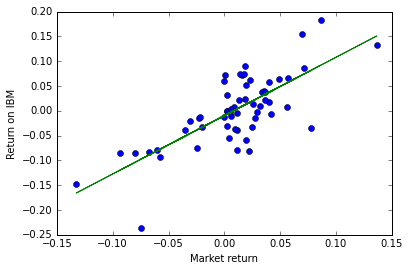# Python application 2: Fama-French regression¶

The purpose of this application is to estimate a Fama-French 3-factor model for a specific corporation. We will study IBM. First, we probably need the usual tools.

In :
import pandas as pd # pandas is excellent for creating and manipulating dataframes, R-style
import numpy as np # great for simulations, we may not use for running regressions here
import matplotlib.pyplot as plt #graphing module with matlab-like properties
%matplotlib inline
import requests # to make requests to webpages (like mine)
import statsmodels.api as sm # module full of standard statistical and econometric models, including OLS and time-series stuff
from IPython.display import Latex # to be able to display latex in python command cells
import getFamaFrenchFactors as gff # a library that downloads FF data nicely, could use datareader for that too
import datetime


Next we build the data we need to run our regressions using various scraping libraries.

In :
# Get five years worth of data for the Fama French 3-factor model (monthly data)

FF3data = gff.famaFrench3Factor(frequency='m') #Datareader could do this too
FF3data=FF3data.tail(60) # keep 5 years of data
FF3data['Month']=FF3data['date_ff_factors'].dt.month
FF3data['Year']=FF3data['date_ff_factors'].dt.year #creates month and year variables for merging below

#import yfinance as yf
#IBM = yf.Ticker("IBM")
#IBMdata=IBM.history(period="5y")

# get 5 years of adjusted close price data for IBM

end=datetime.datetime.today()
IBMdata=DataReader('IBM', 'yahoo', '2015-01-01', end)['Adj Close'] # (at least) five years worth of IBM data
IBMdata=IBMdata.resample('M').last() #only keep the last observation of every month
IBMdataF=IBMdata.to_frame() # converts the series format to a frame format which makes manipulations easier

IBMdataF['Year']=IBMdataF.index.year
IBMdataF['Month']=IBMdataF.index.month

# now we merge our two datasets into one, using year and month as the merging variables

datanow=pd.merge(
IBMdataF,
FF3data,
left_on=['Year','Month'],
right_on=['Year','Month'])

# finally we create IBM's monthly return data from the adjusted price series
# Note the use of panda's shift operator to create a lag variable

datanow.sort_values(['date_ff_factors'],axis=0, ascending=False, inplace=True)

datanow['rIBM']=(datanow['IBM']/datanow.IBM.shift(-1)-1) #computes monthly return based on adjusted series for IBM
datanow['Mkt']=datanow['Mkt-RF']+datanow['RF'] # this the market return, we will use that below
datanow.dropna(subset=['rIBM'], inplace=True) # drop the entries with missing returns (missing due to lag operator)


Now let's look at our data a bit to make sure it all looks good.

In :
datanow[0:4]

Out:
IBM Year Month date_ff_factors Mkt-RF SMB HML RF rIBM Mkt
59 119.209145 2020 6 2020-06-30 0.0245 0.0256 -0.0203 0.0001 -0.033066 0.0246
58 123.285767 2020 5 2020-05-31 0.0558 0.0247 -0.0495 0.0001 0.008084 0.0559
57 122.297081 2020 4 2020-04-30 0.1365 0.0278 -0.0127 0.0000 0.131885 0.1365
56 108.047272 2020 3 2020-03-31 -0.1339 -0.0516 -0.1412 0.0012 -0.147676 -0.1327

Next we plot IBM's monthly return vs the market and fit a capm line. Here we estimate IBM's CAPM beta to be around $1.18$.

In :
datanow.sort_values(['date_ff_factors'],axis=0, ascending=False, inplace=True)

x=datanow['Mkt']
y=datanow['rIBM']

fig, ax = plt.subplots()
plt.plot(x, y, 'o') # each dot is a given month
ax.set_ylabel('Return on IBM')
ax.set_xlabel('Market return')

m, b = np.polyfit(x, y, 1)  # fit the best possible line, beta is the slope of that line
plt.plot(x, m*x + b)  # draw the line on our chart

print('Our estimate of IBMs (CAPM) beta is %.3f' %m)

plt.show() # show our work

Our estimate of IBMs (CAPM) beta is 1.176Instead of taking the quick route above, we can also run a proper CAPM regression. For that we need the excess return on IBM and then we need to estimate the following model: $$r^{IBM}_t-r^F_t= \alpha + \beta \left(r^{S\&P}_t-r^F_t\right)+ \epsilon_t.$$ If CAPM holds, $\alpha$ should estimate to a number that is not statistically different from zero. We will need to create a few variables first.

As shown below, we get about the same estimate of $\beta$ as with the quick route (that's because $r^F$ shows little variability so it is as if it were not in the regression at all) and we do get a statistically insignificant $\alpha.$

In :
datanow['rIBM-rF']=datanow['rIBM']-datanow['RF']

y=datanow['rIBM-rF']
x=datanow['Mkt-RF']
x=sm.add_constant(x) # we run a standard OLS with constant
mod=sm.OLS(y,x)
res=mod.fit()
res.summary()

Out:
Dep. Variable: R-squared: rIBM-rF 0.559 OLS 0.551 Least Squares 72.11 Thu, 27 Aug 2020 1.05e-11 16:23:11 97.047 59 -190.1 57 -185.9 1 nonrobust
coef std err t P>|t| [0.025 0.975] -0.0095 0.006 -1.512 0.136 -0.022 0.003 1.1743 0.138 8.492 0.000 0.897 1.451
 Omnibus: Durbin-Watson: 4.536 2.223 0.104 3.544 -0.494 0.17 3.684 22.4

Warnings:
 Standard Errors assume that the covariance matrix of the errors is correctly specified.

Now we want to estimate a 3-factor Fama-French model for IBM: $$\left(r^{IBM}_t-r^F_t\right)= \alpha + \beta_{Market} \left(r^{Market}_t-r^F_t\right) + \beta_{HML} r^{HML}_t + \beta_{SMB} r^{SMB}_t + \epsilon_t$$ where $\epsilon$ is an error term with all the standard properties. The python for this and the results follow. Only the CAPM beta is significant. Its size changes ever so slightly (though not significantly) which owes to the different specification and, more so, the fact that Fama-French's market portfolio is bigger than just the SP500.

In :
X=datanow[['Mkt-RF','SMB','HML']]
X=sm.add_constant(X) # here we're building our right-hand side variables
y=datanow['rIBM']

mod=sm.OLS(y,X)
res=mod.fit()
res.summary()

Out:
Dep. Variable: R-squared: rIBM 0.557 OLS 0.533 Least Squares 23.07 Thu, 27 Aug 2020 8.44e-10 16:23:11 96.985 59 -186.0 55 -177.7 3 nonrobust
coef std err t P>|t| [0.025 0.975] -0.0088 0.007 -1.314 0.194 -0.022 0.005 1.1698 0.158 7.420 0.000 0.854 1.486 0.0335 0.289 0.116 0.908 -0.545 0.612 -0.0330 0.201 -0.165 0.870 -0.435 0.369
 Omnibus: Durbin-Watson: 3.782 2.21 0.151 2.807 -0.45 0.246 3.575 47.4

Warnings:
 Standard Errors assume that the covariance matrix of the errors is correctly specified.